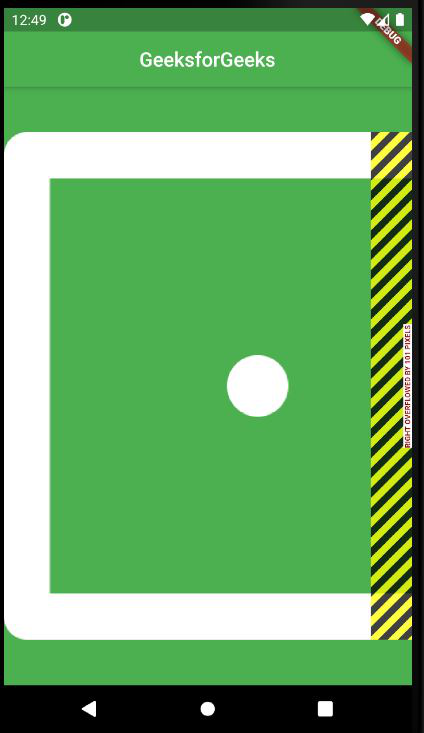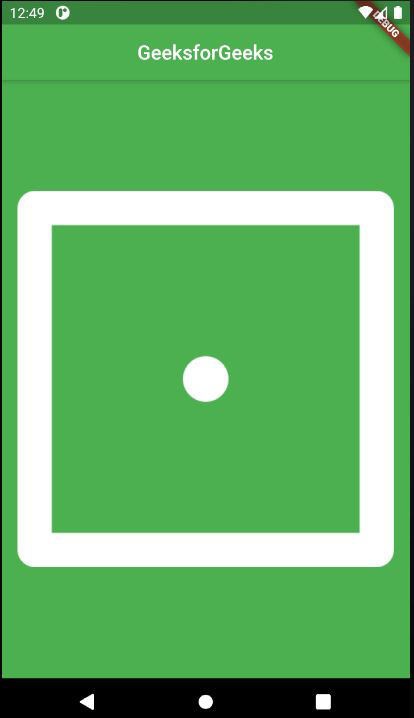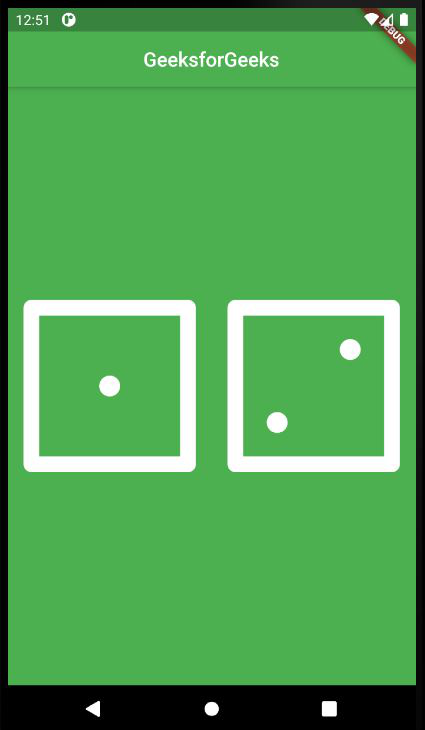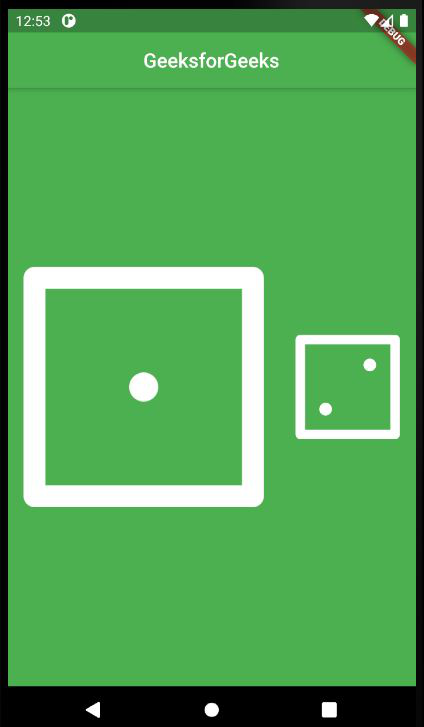Open In App
Related Articles
• Write an Interview Experience

# Expanded Class in Flutter

When we create any child of a row or column we provide the size of the widget according to the screen size but sometimes when we provide more size of child as compared to screen size we get a warning and our widget goes out of the screen for resolving this we put a child of a row or column in an expanded widget so that the child occupies only the available space along the main axis. When we create multiple children then the available space between the children will divide according to the flex factor. An expanded widget contains only the stateful widget or stateless widget not other kinds of widgets like RenderObjectWidgets.

```Syntax:
Expanded(
{
Key key,
int flex: 1,
@required Widget child,
}
)```

### Properties of Expanded Class:

• child: This is the widget present below the expanded class
• Flex factor: Flex factor is the ratio in which available space is divided among the children to occupy the main axis. If the flex factor is zero or NULL the child will allocate its size on its own.
• Key: It manages how one widget is replaced by another widget
• FlexFit: It defines how flexible one widget to acquire available space.

Example:

## Dart

 `import ``'package:flutter/material.dart'``;` `void` `main() {``  ``return` `runApp(``    ``MaterialApp(``      ``home: Scaffold(``        ``backgroundColor: Colors.green,` `        ``// App Bar is used to create``        ``// a bar to give a title for our app``        ``appBar: AppBar(``          ``centerTitle: ``true``,``          ``title: ``const` `Text(``            ``'GeeksforGeeks'``,` `            ``// TextStyle is a class``            ``// where we can modify our text``            ``style: TextStyle(``              ``//To assign the color to our text``              ``color: Colors.green,``            ``), ``//Text Style``          ``), ``//Text` `          ``// backgroundColor is used to change``          ``// the color of our app bar background``          ``backgroundColor: Colors.white,``        ``), ``//AppBar` `        ``// Calling the function DicePage()``        ``body: ``const` `DicePage(),``      ``), ``//Scaffold``    ``), ``//Material App``  ``);``}` `class` `DicePage extends StatefulWidget {``  ``const` `DicePage({Key? key}) : super(key: key);` `  ``@override``  ``// ignore: library_private_types_in_public_api``  ``_DicePageState createState() => _DicePageState();``}` `class` `_DicePageState extends State {``  ``@override``  ``Widget build(BuildContext context) {``    ``return` `Center(``      ``//Here we are using the row.``      ``// Instead of row we can also use``      ``// column only the alignment of icon``      ``// will change and other function remains same``      ``child: Row(``        ``children: [``          ``Expanded(``            ``// FlatButton is used to make``            ``// are image as a button which we can press.``            ``child: TextButton(``              ``child: Container(``                ``color: Colors.green,``                ``padding: ``const` `EdgeInsets.all(14),``                ``child: Image.asset(``'image/dicel.png'``),``              ``),``              ``onPressed: () {},``            ``),` `            ``// FlatButton is depreacted and should not be use``            ``// We can use TextButton instead of FlatButton` `            ``// child: FlatButton(``            ``//   //Image.asset is used to import the image``            ``//   // from our project files only in brackets``            ``//   // we are providing the name of our image.``            ``//   child: Image.asset('images/dice1.png'),``            ``// ), //flat button``          ``), ``//Expanded``        ``], ``//``      ``), ``//Row``    ``); ``//center``  ``}``}`

Output:

In the below image the image of dice goes out of the screen because the required size for the image is more than the screen size.Without using Expanded class

We have used the Expanded class to make our image more flexible so that it can fit the screen size.Image after using Expanded class

By using the Expanded class our image has fitted to available space only.

Example: For Inserting Multiple images

## Dart

 `import ``'package:flutter/material.dart'``;` `void` `main() {``  ``return` `runApp(``    ``MaterialApp(``      ``home: Scaffold(``        ``backgroundColor: Colors.green,` `        ``// App Bar is used to create``        ``// a bar to give a title for our app``        ``appBar: AppBar(``          ``centerTitle: ``true``,``          ``title: ``const` `Text(``            ``'GeeksforGeeks'``,` `            ``// TextStyle is a class``            ``// where we can modify our text``            ``style: TextStyle(``              ``//To assign the color to our text``              ``color: Colors.green,``            ``), ``//Text Style``          ``), ``//Text` `          ``// backgroundColor is used to``          ``// change the color of our``          ``// app bar background``          ``backgroundColor: Colors.white,``        ``), ``//AppBar` `        ``//Calling the function DicePage()``        ``body: ``const` `DicePage(),``      ``), ``//Scaffold``    ``), ``//Material App``  ``);``}` `class` `DicePage extends StatefulWidget {``  ``const` `DicePage({Key? key}) : super(key: key);` `  ``@override``  ``// ignore: library_private_types_in_public_api``  ``_DicePageState createState() => _DicePageState();``}` `class` `_DicePageState extends State {``  ``@override``  ``Widget build(BuildContext context) {``    ``return` `Center(``      ``// Here we are using the row.``      ``// Instead of row we can also use column only``      ``// the alignment of icon will change and other``      ``// function remains same``      ``child: Row(``        ``children: [``          ``// For Image 1``          ``Expanded(``            ``// Flex is used to allocate``            ``// available space in the ratio``            ``// of one child to another child``            ``// it can be any value.``            ``flex: 2,` `            ``child: TextButton(``              ``child: Container(``                ``color: Colors.green,``                ``padding: ``const` `EdgeInsets.all(14),``                ``child: Image.asset(``'image/dicel1.png'``),``              ``),``              ``onPressed: () {},``            ``),` `            ``// FlatButton is depreacted and should not be use``            ``// We can use TextButton instead of FlatButton` `            ``// FlatButton is used to make``            ``// are image as a button which we can press.` `            ``// child: FlatButton(``            ``//   // Image.asset is used to import``            ``//   // the image from our project files only``            ``//   // in brackets we are providing the name of our image.``            ``//    child: Image.asset('images/dice1.png'),``            ``//  ),  //flat button``          ``),``          ``// For image 2.``          ``Expanded(``            ``child: TextButton(``              ``child: Container(``                ``color: Colors.green,``                ``padding: ``const` `EdgeInsets.all(14),``                ``child: Image.asset(``'image/dicel2.png'``),``              ``),``              ``onPressed: () {},``            ``),` `            ``// FlatButton is depreacted and should not be use``            ``// We can use TextButton instead of FlatButton` `            ``//  child: FlatButton(``            ``//    child: Image.asset('images/dice2.png'),``            ``//  ),  //flat button``          ``), ``//Expanded``        ``], ``//``      ``), ``//Row``    ``); ``//center``  ``}``}`

Output:

In this, we have inserted the multiple-image of dice. By default, the flex size is 1, but we can change it to our requirement.Multiple Images

This Flex factor is set to 2 and image 1 is twice the size of image two.Multiple images with Flex factor